D-error is a measure that quantifies how good or bad a design is at extracting information from respondents in an experiment. A lower D-error indicates a better design. A design that minimizes D-error is considered D-optimal. Many other related measures exist that also serve this purpose, such as D-optimality. This article describes computing D-error, Bayesian D-error, and D-efficiency.

## Computing D-error

I'll show you how to compute D-error to assess the quality of your experiment. There are many different types of experiments. One type is a choice experiment, which represents a design with a table where each row corresponds to a choice alternative and the columns correspond to the version,  task, question, alternative, and attributes. I've shown you an example of a design below:

1111121
1112212
1221122
1222211
1331221
1332112
2411222
2412111
2521222
2522111
2631121
2632212

To compute D-error, first encode the levels of the attributes in the design using dummy coding (or alternatively effects-type coding) and split the encoded design by questions (suppose there are Q of them) to create a set of matrices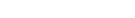In addition, you need to make a prior assumption regarding the respondent parameters. The different possible assumptions lead to three variants of the D-error. The first is the Dp-error, which assumes that all respondent parameters are given by a parameter vector β . The multinomial logit probability that a respondent chooses alternative 𝑖 in question 𝑞 is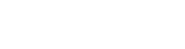where J is the number of alternatives per question and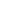is a vector of length J.

We first construct the Fisher information matrix M using the formula below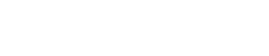where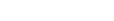Dp-error is a function of the determinant of the information matrix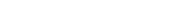where K is the number of parameters in β.

## D0-error

The simpler D0-error assumes that respondents have no preference for any of the attribute levels, which is equivalent to assuming that β=0. The logit probabilities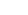become 1/J for all questions and alternatives. The information matrix formula in this case reduces to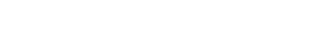where 1J is a vector of J ones. Apart from this difference, the D0-error is computed using the same function of the determinant as for DP-error. A design created by optimizing D0-error is known as a utility-neutral design.

## DB-error

Lastly, DB-error assumes that respondent parameters are distributed according to a probability distribution, which is often a multivariate normal distribution with a diagonal covariance matrix.

DB-error is simply the integral of DP-error over the prior distribution of respondent parameters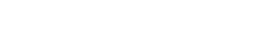where π(β) denotes the density of the prior distribution at β. A design created by optimizing DB-error is also known as a Bayesian design.

## D-efficiency

D-efficiency is a relative measure based on comparing one design X against a benchmark design X*. You can compute D-efficiency for any of the three D-errors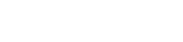If the benchmark design has a lower D-error, the D-efficiency has a range from 0 to 1, and it will be close to 1 if the design is close to the benchmark.

Ready to find out more about D-Errors and assessing the quality of your design? Check out the Displayr blog